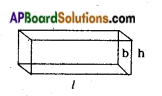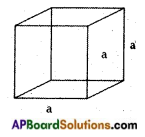Students can go through AP Board 8th Class Maths Notes Chapter 14 Surface Areas and Volume to understand and remember the concepts easily.

## AP State Board Syllabus 8th Class Maths Notes Chapter 14 Surface Areas and Volume

→ If l, b, h are-the dimensions of cuboid, then:(i) its lateral surface area is 2h (l+ b)
(ii) its total surface area is 2 (lb + bh + hl)
(iii) its volume is l × b × h

→ Lateral surface area of a cube is 4a2→ Total surface area of a cube is 6a2→ Volume of a cube is side × side × side = a3

→ 1 cm3 = 1 ml

→ 1 l = 1000 cm3

→ 1 m3 = 1000000 cm3 = 1000 l = 1 kl (kilolitre)